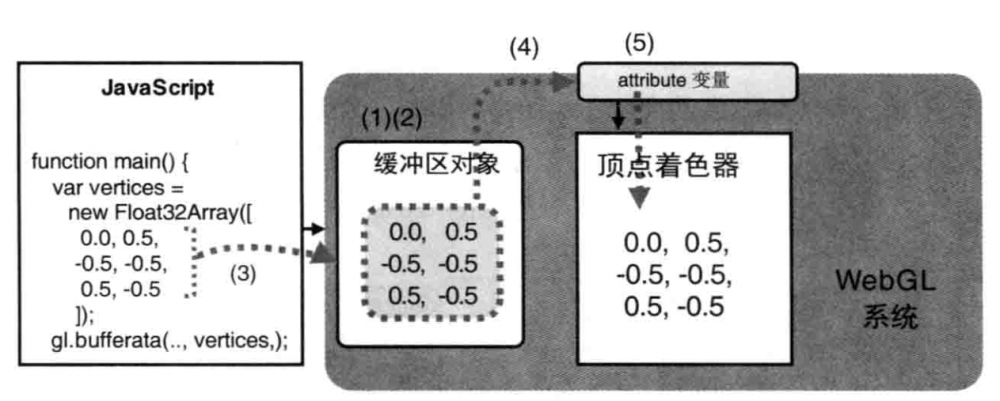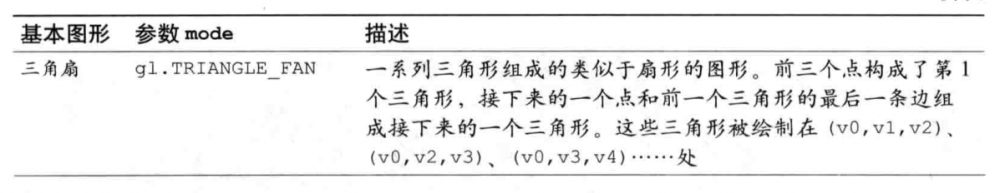# 2. 示例：绘制三角形

## 1) HelloTriangle.html

``````<!DOCTYPE html>
<html lang="en">
<meta charset="utf-8" />
<title>Hello Triangle</title>

<canvas id="webgl" width="400" height="400">
Please use a browser that supports "canvas"
</canvas>

<script src="../lib/webgl-utils.js"></script>
<script src="../lib/webgl-debug.js"></script>
<script src="../lib/cuon-utils.js"></script>
<script src="HelloTriangle.js"></script>
</body>
</html>
``````

## 2) HelloTriangle.js

``````// 顶点着色器程序
'attribute vec4 a_Position;/n' + // attribute variable
'void main() {/n' +
'  gl_Position = a_Position;/n' + // Set the vertex coordinates of the point
'}/n';

// 片元着色器程序
'precision mediump float;/n' +
'uniform vec4 u_FragColor;/n' +  // uniform変数
'void main() {/n' +
'  gl_FragColor = vec4(1.0, 0.0, 0.0, 1.0);/n' +
'}/n';

function main() {
// 获取 <canvas> 元素
var canvas = document.getElementById('webgl');

// 获取WebGL渲染上下文
var gl = getWebGLContext(canvas);
if (!gl) {
console.log('Failed to get the rendering context for WebGL');
return;
}

// 初始化着色器
return;
}

// 设置顶点位置
var n = initVertexBuffers(gl);
if (n < 0) {
console.log('Failed to set the positions of the vertices');
return;
}

// 指定清

gl.clearColor(0.0, 0.0, 0.0, 1.0);

// 清空<canvas>
gl.clear(gl.COLOR_BUFFER_BIT);

// 绘制三角形
gl.drawArrays(gl.TRIANGLES, 0, 3);
}

function initVertexBuffers(gl) {
var vertices = new Float32Array([
0, 0.5,   -0.5, -0.5,   0.5, -0.5
]);
var n = 3; // 点的个数

// 创建缓冲区对象
var vertexBuffer = gl.createBuffer();
if (!vertexBuffer) {
console.log('Failed to create the buffer object');
return -1;
}

// 将缓冲区对象绑定到目标
gl.bindBuffer(gl.ARRAY_BUFFER, vertexBuffer);
// 向缓冲区对象写入数据
gl.bufferData(gl.ARRAY_BUFFER, vertices, gl.STATIC_DRAW);

var a_Position = gl.getAttribLocation(gl.program, 'a_Position');
if (a_Position < 0) {
console.log('Failed to get the storage location of a_Position');
return -1;
}
// 将缓冲区对象分配给a_Position变量
gl.vertexAttribPointer(a_Position, 2, gl.FLOAT, false, 0, 0);

// 连接a_Position变量与分配给它的缓冲区对象
gl.enableVertexAttribArray(a_Position);

return n;
}
``````

## 3) 缓冲区对象

``````var vertices = new Float32Array([
0, 0.5,   -0.5, -0.5,   0.5, -0.5
]);
``````

### (1) 创建缓冲区对象(gl.createBuffer())

``````  // 创建缓冲区对象
var vertexBuffer = gl.createBuffer();
if (!vertexBuffer) {
console.log('Failed to create the buffer object');
return -1;
}
``````

WebGL通过gl.createBuffer()来创建缓冲区对象，它告诉WebGL系统，开辟显存空间接受内存传输过来的数据。其函数的具体说明如下：### (2) 绑定缓冲区对象(gl.bindBuffer())

``````  // 将缓冲区对象绑定到目标
gl.bindBuffer(gl.ARRAY_BUFFER, vertexBuffer);
``````### (3) 将数据写入缓冲区对象(gl.bufferData())

``````  // 向缓冲区对象写入数据
gl.bufferData(gl.ARRAY_BUFFER, vertices, gl.STATIC_DRAW);
``````### (4) 将缓冲区对象分配给attribute变量(gl.vertexAttribPointer())

``````  var a_Position = gl.getAttribLocation(gl.program, 'a_Position');
if (a_Position < 0) {
console.log('Failed to get the storage location of a_Position');
return -1;
}
// 将缓冲区对象分配给a_Position变量
gl.vertexAttribPointer(a_Position, 2, gl.FLOAT, false, 0, 0);
``````### (5) 开启attribute变量(gl.enableVertexAttribArray())

``````  // 连接a_Position变量与分配给它的缓冲区对象
gl.enableVertexAttribArray(a_Position);
``````## 4) 基本图形绘制

``````  // 绘制三角形
gl.drawArrays(gl.TRIANGLES, 0, 3);
``````

awArrays()进行绘制的，其具体的函数说明如下：# 3. 结果# 4. 参考

WEBGL学习网（WebGLStudy.COM）专注提供WebGL 、ThreeJS、BabylonJS等WEB3D开发案例源码下载。

1. 本站部分资源来源于用户上传和网络，如有侵权请邮件联系站长：1218436398@qq.com！我们将尽快处理。
2. 分享目的仅供大家学习和交流，您必须在下载后24小时内删除！
3. 不得使用于非法商业用途，不得违反国家法律。否则后果自负！
4. 本站提供的源码、模板、插件等等其他资源，都不包含技术服务请大家谅解！
5. 如有链接无法下载、失效或广告，请联系管理员处理！
6. 本站资源打赏售价用于赞助本站提供的服务支出（包括不限服务器、网络带宽等费用支出）！
7.欢迎加QQ群学习交流：549297468 ，或者搜索微信公众号：WebGL学习网
WEBGL学习网 » WebGL基础入门教程(三)：绘制一个三角形(缓冲区对象)